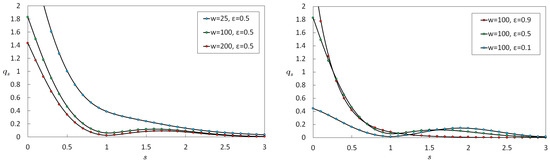Next Article in Journal
Low-Momentum Pion Enhancement from Schematic Hadronization of a Gluon-Saturated Initial State
Next Article in Special Issue
Strong Effective Coupling, Meson Ground States, and Glueball within Analytic Confinement
Previous Article in Journal
Causality and Renormalization in Finite-Time-Path Out-of-Equilibrium ϕ3 QFT
Previous Article in Special Issue
Some Recent Results on High-Energy Proton Interactions
Due to scheduled maintenance work on our core network, there may be short service disruptions on this website between 16:00 and 16:30 CEST on September 25th.
Article

# S-Matrix of Nonlocal Scalar Quantum Field Theory in Basis Functions Representation

1
Moscow Institute of Physics and Technology (MIPT), Institutskiy Pereulok 9, 141701 Dolgoprudny, Russia
2
Dukhov Research Institute of Automatics (VNIIA), Sushchevskaya 22, 127055 Moscow, Russia
*
Authors to whom correspondence should be addressed.
Particles 2019, 2(1), 103-139; https://doi.org/10.3390/particles2010009
Received: 18 December 2018 / Revised: 1 February 2019 / Accepted: 12 February 2019 / Published: 19 February 2019
Nonlocal quantum theory of a one-component scalar field in D-dimensional Euclidean spacetime is studied in representations of $S$ -matrix theory for both polynomial and nonpolynomial interaction Lagrangians. The theory is formulated on coupling constant g in the form of an infrared smooth function of argument x for space without boundary. Nonlocality is given by the evolution of a Gaussian propagator for the local free theory with ultraviolet form factors depending on ultraviolet length parameter l. By representation of the $S$ -matrix in terms of abstract functional integral over a primary scalar field, the $S$ form of a grand canonical partition function is found. By expression of $S$ -matrix in terms of the partition function, representation for $S$ in terms of basis functions is obtained. Derivations are given for a discrete case where basis functions are Hermite functions, and for a continuous case where basis functions are trigonometric functions. The obtained expressions for the $S$ -matrix are investigated within the framework of variational principle based on Jensen inequality. Through the latter, the majorant of $S$ (more precisely, of $− ln S$ ) is constructed. Equations with separable kernels satisfied by variational function q are found and solved, yielding results for both polynomial theory $φ 4$ (with suggestions for $φ 6$ ) and nonpolynomial sine-Gordon theory. A new definition of the $S$ -matrix is proposed to solve additional divergences which arise in application of Jensen inequality for the continuous case. Analytical results are obtained and numerically illustrated, with plots of variational functions q and corresponding majorants for the $S$ -matrices of the theory. For simplicity of numerical calculation, the $D = 1$ case is considered, and propagator for free theory G is in the form of Gaussian function typically in the Virton–Quark model, although the obtained analytical inferences are not, in principle, limited to these particular choices. Formulation for nonlocal QFT in momentum k space of extra dimensions with subsequent compactification into physical spacetime is discussed, alongside the compactification process. View Full-Text
Show FiguresFigure 1

MDPI and ACS Style

Chebotarev, I.V.; Guskov, V.A.; Ogarkov, S.L.; Bernard, M. S-Matrix of Nonlocal Scalar Quantum Field Theory in Basis Functions Representation. Particles 2019, 2, 103-139. https://doi.org/10.3390/particles2010009

AMA Style

Chebotarev IV, Guskov VA, Ogarkov SL, Bernard M. S-Matrix of Nonlocal Scalar Quantum Field Theory in Basis Functions Representation. Particles. 2019; 2(1):103-139. https://doi.org/10.3390/particles2010009

Chicago/Turabian Style

Chebotarev, Ivan V., Vladislav A. Guskov, Stanislav L. Ogarkov, and Matthew Bernard. 2019. "S-Matrix of Nonlocal Scalar Quantum Field Theory in Basis Functions Representation" Particles 2, no. 1: 103-139. https://doi.org/10.3390/particles2010009

Find Other Styles

1